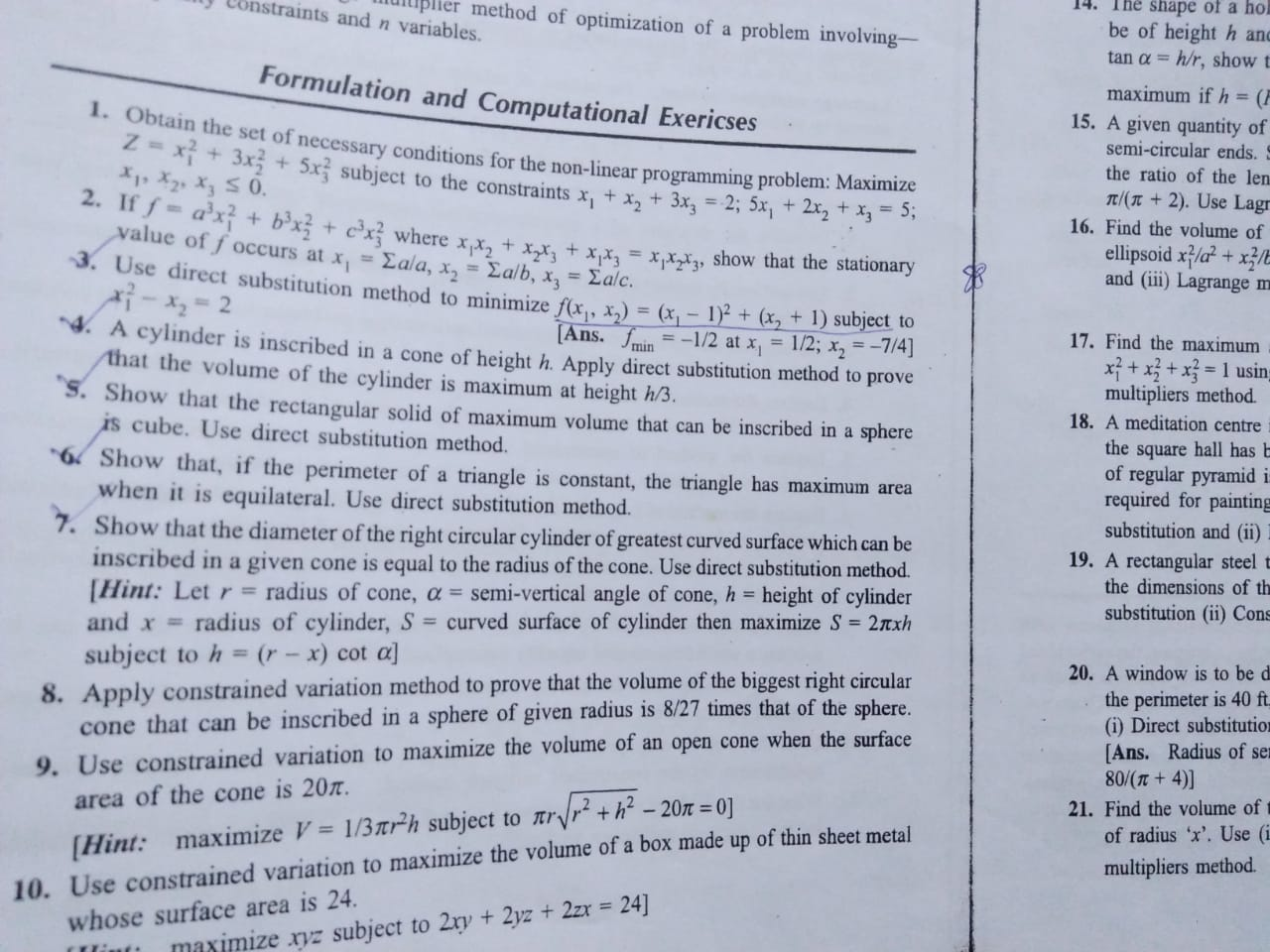# 14· The shape of a holbe of height h andtan α-: hh, show tmaximum if h = (15. A given quantity ofsemi-circular ends. Sthe ratio of the lenm(π + 2). Use Lagr16. Find the volume ofellipsoid x2は x1and (iii) Lagrange mlipler method of optimization of a problem involving-Constraints and n variables.Formulation and Computational Exericsesain the set of necessary conditions for the non-linear programming problem: Maximize2. If f = a3xf + b3xf + ex where x1x2 + x25 + xM" xix-X3, show that the stationary3. Use direct substitution method to minimize f(x, x)+() subject tocylinder is inscribed in a cone of height h. Apply direct substitution method to proveS. Show that the rectangular solid of maximum volume that can be inscribed in a sphere6 Show that, if the perimeter of a triangle is constant, the triangle has maximum area3x5xj subject to the constraintsxx2 3x 2; 5x12x2value off occurs at x," Σα/a, X2 = Σα/b, x,-Σα/c.4. A17. Find the maximumns. Jminat the volume of the cylinder is maximum at height hsis cube. Use direct substitution method.when it is equilateral. Use direct substitution method.multipliers method.18. A meditation centrethe square hall has bof regular pyramid irequired for paintingsubstitution and (ii)7. Show that the diameter of the right circular cylinder of greatest curved surface which can beinscribed in a given cone is equal to the radius of the cone. Use direct substitution method.[Hint: Let r = radius of cone, α semi-vertical angle of cone, h = height of cylinderand x = radius of cylinder, S-curved surface of cylinder then maximize S= 2TXh19. A rectangular steel tthe dimensions of thsubstitution (ii) Conssubject toh(r-x) cot a]20. A window is to be dApply constrained variation method to prove that the volume of the biggest right circularcone that can be inscribed in a sphere of given radius is 8/27 times that of the sphere.9. Use constrained variation to maximize the volume of an open cone when the surfacethe perimeter is 40 ft(i) Direct substitutior[Ans. Radius of se80/(+area of the cone is 20π.[Hint: maximize V = 1/3㎡h subject to πrVr2 + h2-20 0]whose surface area is 24.21. Find the volume of tof radius ‘x'. Use (imultipliers method.10.Use constrained variation to maximize the volume of a box made up of thin sheet metalmaximize rvz subject to 2n, + 2)z + 2zr = 24]

Question
4 views

I need you to solve question no 5 onlyhelp_outlineImage Transcriptionclose14· The shape of a hol be of height h and tan α-: hh, show t maximum if h = ( 15. A given quantity of semi-circular ends. S the ratio of the len m(π + 2). Use Lagr 16. Find the volume of ellipsoid x2は x1 and (iii) Lagrange m lipler method of optimization of a problem involving- Constraints and n variables. Formulation and Computational Exericses ain the set of necessary conditions for the non-linear programming problem: Maximize 2. If f = a3xf + b3xf + ex where x1x2 + x25 + xM" xix-X3, show that the stationary 3. Use direct substitution method to minimize f(x, x)+() subject to cylinder is inscribed in a cone of height h. Apply direct substitution method to prove S. Show that the rectangular solid of maximum volume that can be inscribed in a sphere 6 Show that, if the perimeter of a triangle is constant, the triangle has maximum area 3x 5xj subject to the constraintsxx2 3x 2; 5x12x2 value off occurs at x," Σα/a, X2 = Σα/b, x,-Σα/c. 4. A 17. Find the maximum ns. Jmin at the volume of the cylinder is maximum at height hs is cube. Use direct substitution method. when it is equilateral. Use direct substitution method. multipliers method. 18. A meditation centre the square hall has b of regular pyramid i required for painting substitution and (ii) 7. Show that the diameter of the right circular cylinder of greatest curved surface which can be inscribed in a given cone is equal to the radius of the cone. Use direct substitution method. [Hint: Let r = radius of cone, α semi-vertical angle of cone, h = height of cylinder and x = radius of cylinder, S-curved surface of cylinder then maximize S= 2TXh 19. A rectangular steel t the dimensions of th substitution (ii) Cons subject toh(r-x) cot a] 20. A window is to be d Apply constrained variation method to prove that the volume of the biggest right circular cone that can be inscribed in a sphere of given radius is 8/27 times that of the sphere. 9. Use constrained variation to maximize the volume of an open cone when the surface the perimeter is 40 ft (i) Direct substitutior [Ans. Radius of se 80/(+ area of the cone is 20π. [Hint: maximize V = 1/3㎡h subject to πrVr2 + h2-20 0] whose surface area is 24. 21. Find the volume of t of radius ‘x'. Use (i multipliers method. 10. Use constrained variation to maximize the volume of a box made up of thin sheet metal maximize rvz subject to 2n, + 2)z + 2zr = 24] fullscreen
check_circle

Step 1

To prove that the volume of a rectangular solid under the given conditions attains its maximum when all the sides are equal (cube)

Step 2

The diagram represents the rectangular box of varying sides L , B and H inscribed inside the sphere of radius R ,say. For varying L,B,H , we obtain different volumes, and we need to show that the maximum volume is attained when all the sides are equal (in other words, the box is a cube)

Step 3

To maximize V  (as a function of the vari...

### Want to see the full answer?

See Solution

#### Want to see this answer and more?

Solutions are written by subject experts who are available 24/7. Questions are typically answered within 1 hour.*

See Solution
*Response times may vary by subject and question.
Tagged in

### Math